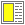# Exercise 3.6 Sublists of a list ***

In this exercise we will program a function front-sublist which returns the first n elements of a list. The signature (the head) of the function should be (front-sublist lst n) where lst is a list and n is a number. As a precondition it can be assumed that lst is a proper list and that n is a non-negative integer. As a postcondition we want to guarantee that the length of the result is n.

As an example

```        (front-sublist '(a b c d e) 3)  =>
(a b c)

(front-sublist '(a b c d e) 6)  =>
ERROR```

First, identify the extreme, border cases and be sure that you know how to handle these. Next, program the function with due respect to both the precondition and the postcondition. Next, test the function carefully in a dialogue with your Scheme system.

Given the function front-sublist we will program the function sublists, which breaks a proper list into a list of sublists of some given size. As an example

```        (sublists '(a b c d e f) 3) =>
((a b c) (d e f))```

Program the function sublists with use of front-sublist. Be careful to prepare you solution by recursive thinking. It means that you should be able to break the overall problem into a smaller problem of the same nature, as the original problem. You should feel free to formulate both preconditions and postconditions of the function sublists, such that the existing function front-sublist fits well.

Hint: The Scheme function list-tail is probably useful when you program the function sublists.

A table can be represented as a list of rows. This is, in fact, the way tables are represented in HTML. The tr tag is used to mark each row; the td tag is used to mark each cell. The table tag is used to mark the overall table. Thus, the list of rows ((a b c) (d e f)) will be marked up as:

```         <table>
<tr> <td>a</td> <td>b</td> <td>c</td> </tr>
<tr> <td>d</td> <td>e</td> <td>f</td> </tr>
</table>```

Write a Scheme function called table-render that takes a list of rows as input (as returned by the function sublists, which we programmed above) and returns the appropriate HTML rendering of the rows. Use the LAML mirror functions table, tr, and td. Be sure to call the LAML function xml-render to see the textual HTML rendering of the result, as opposed to LAML's internal representation.

Notice: During the course we will see better and better ways to program table-render. Nevertheless, it is a good idea already now to program a first version of it.

There is no solution to this exercise